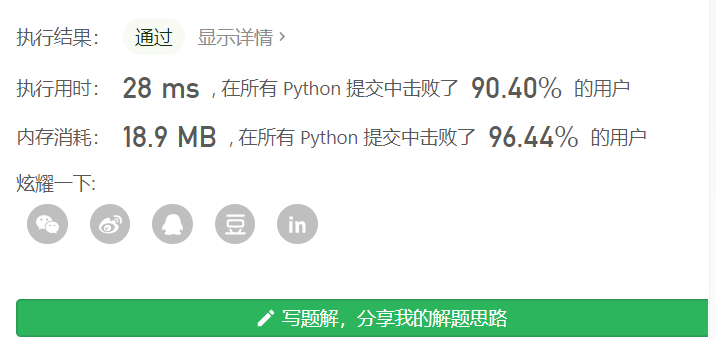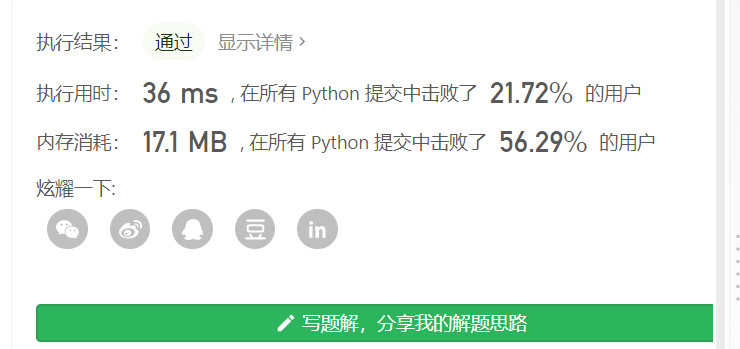## 一些算法题的简单总结（python实现）

2021/2/8 15:55:46 文章标签: 测试文章如有侵权请发送至邮箱809451989@qq.com投诉后文章立即删除

# 剑指 Offer 03. 数组中重复的数字

class Solution(object):
def findRepeatNumber(self, nums):
"""
:type nums: List[int]
:rtype: int
根据该数字的数值找到对应的下标位置，如果该位置的数字的数值等于其下标
那么这个数字是重复的
"""

i=0
while i<len(nums):
if nums[i]==i:
i+=1
else:
if nums[nums[i]]==nums[i]:
return nums[i]
else:
nums[nums[i]],nums[i]=nums[i],nums[nums[i]]# 剑指 Offer 04. 二维数组中的查找

• 如果从左上角开始查找，假如target大于左上角的值，那么target既有可能在右边也有可能在下边，假如target小于左上角的值，那么返回False
• 如果从左下角开始查找，假如target大于左下角的值，那么target只能在右边，假如target小于左下角的值，那么target只能在上边，直到找到右上角，返回False
• 如果从右上角开始查找，假如target大于右上角的值，那么target只能在下边，假如target小于右上角的值，那么target只能在左边，直到找到左下角，返回False
• 如果从右下角开始查找，假如target大于右下角的值，那么返回False，假如target小于右下角的值，那么target既有可能在左边也有可能在上边

class Solution(object):
def findNumberIn2DArray(self, matrix, target):
"""
:type matrix: List[List[int]]
:type target: int
:rtype: bool
如果从左上角开始查找，假如target大于左上角的值，那么target既有可能在右边也有可能在下边，假如target小于左上角的值，那么返回False
如果从左下角开始查找，假如target大于左下角的值，那么target只能在右边，假如target小于左下角的值，那么target只能在上边，直到找到右上角，返回False
如果从右上角开始查找，假如target大于右上角的值，那么target只能在下边，假如target小于右上角的值，那么target只能在左边，直到找到左下角，返回False
如果从右下角开始查找，假如target大于右下角的值，那么返回False，假如target小于右下角的值，那么target既有可能在左边也有可能在上边

所以我们选择从左下角或者右上角开始查找
"""
if matrix==[]:
return False
n=len(matrix)
m=len(matrix)
if m==0:
return False
if target<matrix or target>matrix[n-1][m-1]:
return False

#从左下角开始查找
i=n-1
j=0
while i>=0 and j<=m-1:
if target==matrix[i][j]:
return True
elif target>matrix[i][j]:
j+=1
else:
i-=1
return False# 剑指 Offer 05. 替换空格

//由于python中的字符串是不能进行赋值操作的，因为python中的字符串被看作是常量，所以下面用C++实现。
class Solution {
public:
string replaceSpace(string s) {

if(s.length()==0){return s;}

int length=s.length();
int blank_nums=0;
for(int i=0;i<length;i++){
if(s[i]==' '){blank_nums++;}
}
int old_i=s.length()-1;
s+=string(blank_nums*2,' ');
//统计s中有多少个空格，为什么是乘以2呢，因我们要将空格替换为%20，所以原始字符串的每一个空格位置要额外加上两个字符的位置才能被替换为%20
//所以新的字符串的长度是原来字符串的长度加上2*空格数目

int i=s.length()-1;
while(old_i>=0)
{
if(s[old_i]!=' '){
s[i--]=s[old_i--];
}
else{
s[i--]='0';
s[i--]='2';
s[i--]='%';
old_i-=1;
}
}
return s;
}
};# 剑指 Offer 06. 从尾到头打印链表

# Definition for singly-linked list.
# class ListNode(object):
#     def __init__(self, x):
#         self.val = x
#         self.next = None

return
else:
#(eg: a=[0,1],b=a)两个变量引用的是同一个内存地址([0,1]的内存地址)，所以在函数中对list修改以后，是直接在实参上修改的

class Solution(object):
"""
:rtype: List[int]
"""
res=[]
return res# 剑指 Offer 07. 重建二叉树

前序遍历 preorder = [3,9,20,15,7]



• 根据preorder知道根节点是3，然后在inorder中找到3的位置
• 找到3的位置之后，知道了左子树是9，右子树是[20,15,7]，然后递归即可
# Definition for a binary tree node.
# class TreeNode(object):
#     def __init__(self, x):
#         self.val = x
#         self.left = None
#         self.right = None

class Solution(object):
def recursiveTree(self,Node,preorder,inorder):
if len(preorder)==0 or len(inorder)==0:
return None
root_val=preorder
Node=TreeNode(root_val)
root_pos=inorder.index(root_val)

Node.left=self.recursiveTree(Node.left,preorder[1:root_pos+1],inorder[:root_pos])
Node.right=self.recursiveTree(Node.right,preorder[root_pos+1:],inorder[root_pos+1:])
return Node

def buildTree(self, preorder, inorder):
"""
:type preorder: List[int]
:type inorder: List[int]
:rtype: TreeNode
根据前序的第一个节点去中序中找到相对应的节点，划分开左右子树
然后递归即可
"""# 剑指 Offer 09. 用两个栈实现队列

class CQueue(object):

def __init__(self):
'''
我们要定义两个list
这两个list只能使用append和pop方法，不能使用索引直接返回
实现思路是：如果有元素要进来，那么就把这些元素压入push_stack中
如果有元素要出去，那么此时要判断pop_stack中是否含有元素
，如果没有那么就把push_stack中的全部元素都pop到pop_stack中，
然后pop_stack.pop就是要弹出的元素，
如果有，那么直接pop_stack.pop
'''
self.push_stack=[]
self.pop_stack=[]

def appendTail(self, value):
"""
:type value: int
:rtype: None
"""
self.push_stack.append(value)

"""
:rtype: int
"""
if self.push_stack==[] and self.pop_stack==[]:
return -1
else:
if self.pop_stack!=[]:
return self.pop_stack.pop()
else:
assert self.push_stack!=[]
while self.push_stack!=[]:
self.pop_stack.append(self.push_stack.pop())
return self.pop_stack.pop()# 剑指 Offer 10- I. 斐波那契数列

class Solution(object):
def fib(self, n):
"""
:type n: int
:rtype: int
"""

if n==0:
return 0
if n==1:
return 1

a=0
b=1
mod=1e9+7
for i in range(2,n+1):
c=a+b
c=int(c%mod)
a,b=b,c

return c# 剑指 Offer 11. 旋转数组的最小数字

class Solution(object):
def sequence_find(self,numbers):
min_val=numbers
for i in range(1,len(numbers)):
if numbers[i]<min_val:
min_val=numbers[i]
return min_val

def minArray(self, numbers):
"""
:type numbers: List[int]
:rtype: int
a=[3,4,5,6,7,8,1,2]#在右边
b=[6,7,8,1,2,3,4,5]#在中间
c=[7,8,1,2,3,4,5,6]#在左边
利用low,high两个指针，我们看到如下规律
if numbers[low]>numbers[high]:
那么说明当前的numbers存在旋转的情况
设temp=numbers[middle]，如果temp的值大于numbers[low],
那么最小值一定在[middle和high之间]
如果temp的值小于numbers[low]，
那么说明此时low和middle之间存在翻转，而且最小值一定在low和middle之间
"""
if len(numbers)==1:
return numbers
low=0
high=len(numbers)-1
if numbers[low]<numbers[high]:
#说明不存在旋转的情况
return numbers[low]

while high-low!=1:
middle=(high+low)//2
if numbers[middle]==numbers[low]==numbers[high]:
#无法判断在哪边
return self.sequence_find(numbers)
if numbers[middle]>=numbers[low]:
assert numbers[middle]>numbers[high] and numbers[low]>=numbers[high]
low=middle
else:
assert numbers[middle]<=numbers[high] and numbers[low]>=numbers[high]
high=middle
if numbers[low]<numbers[high]:
return numbers[low]
else:
return numbers[high]# 剑指 Offer 12. 矩阵中的路径

[["a","b","c","e"],
["s","b","c","s"],
["a","d","d","e"]]


• 发现第一个单词就不匹配，返回False
• 然后从开始，第一个单词匹配b，接下来就是从b的四个方向开始搜索，首先搜索左边
• 发现a不匹配c，接下来搜索右边
• 然后找到c，接下来就是从c的四个方向的开始搜索，此时b,c位置已经被标记为访问过，所以c的左边不能查找
• 发现c的四周没有匹配下一个字符d的，那么回溯到b，此时b还有上下两个方向没有被搜索
• 但是上下两个方向都不匹配c，递归结束，返回False
• 然后接着按照上述步骤重复的遍历数组中的每一个元素，看看从这个元素开始能不能找到"bcd"这个路径
• 假如我们现在从第二行第二列的b开始，仍然是递归左边，发现‘a’不匹配’c’，递归上下也都发现不匹配’c’，然后递归到右边
• 从’c’开始，发现下边的元素’d’正好匹配，然后递归到下面，此时完整的匹配’bcd’，返回True即可
def find_path(board,word,visited,index,position):
i,j=position
if index==len(word):
return True

if i>=0 and j>=0 and i<len(board) and j<len(board) and \
board[i][j]==word[index] and visited[i][j]==False:
visited[i][j]=True
index+=1

left_find=find_path(board,word,visited,index=index,position=(i,j-1))
right_find=find_path(board,word,visited,index=index,position=(i,j+1))
up_find=find_path(board,word,visited,index=index,position=(i-1,j))
down_find=find_path(board,word,visited,index=index,position=(i+1,j))

found=left_find or right_find or up_find or down_find
if found==False:
visited[i][j]=False
index-=1
return False
else:
return True
else:
return False

class Solution(object):
def exist(self, board, word):
"""
:type board: List[List[str]]
:type word: str
:rtype: bool
采用回溯法，需要注意的是路径可以从任意一点开始，所以需要遍历矩阵中的每一个元素
直到找到路径位置

在查找路径的过程中，需要设置一个visited数组用来记录每一个节点是否已经访问过
当某一个节点的上下左右四个方向都不能匹配下一个字符的时候，那么就回溯到这个节点的上一个节点
并且将这个节点的visited重置为False
"""
visited=[]
for i in range(len(board)):
visited.append([False]*len(board[i]))

for i in range(len(board)):
for j in range(len(board[i])):
if find_path(board,word,visited,index=0,position=(i,j)):
return  True
return False# 剑指 Offer 13. 机器人的运动范围

def get_position_sum(i,j):
i_list=list(str(i))
j_list=list(str(j))
res=0
for ii in i_list:
res+=int(ii)
for jj in j_list:
res+=int(jj)
return res

def recursive_moving(m,n,k,i,j,count_nums_list,visited):
if i>=0 and j>=0 and i<=m-1 and j<=n-1 and get_position_sum(i,j)<=k and visited[i][j]==False:
count_nums_list+=1
visited[i][j]=True
recursive_moving(m,n,k,i+1,j,count_nums_list,visited)
recursive_moving(m,n,k,i,j+1,count_nums_list,visited)

class Solution(object):
def movingCount(self, m, n, k):
"""
:type m: int
:type n: int
:type k: int
:rtype: int
回溯法，机器人从左上角开始移动，其实本题是不需要考虑向上或者向左的情况
因为机器人就是从左向右，从上到下的移动的。
每次移动到一个位置，判断这个位置的下方和右方是否可以到达，
如果可以，则递归到右侧或者下侧（要利用一个visited数组记录每一个位置是否已经被访问过了，防止重复访问）
"""
count_nums_list=
visited=[[False]*n for _ in range(m)]
recursive_moving(m,n,k,0,0,count_nums_list,visited)#利用列表是因为列表可以在函数内部修改参数
return count_nums_list# 剑指 Offer 14- I. 剪绳子

class Solution(object):
def cuttingRope(self, n):
"""
:type n: int
:rtype: int
求最大值这种，涉及到最优解，而且一个绳子剪成两段之后，这两段的每一段
又是同样的分析思路，所以可以考虑用动态规划，因为子问题是重复的，而且子问题之间是重叠的，
每一个子问题的性质一样，只是规模变小，这些都符合动态规划的性质。
状态转移方程： dp[i]=dp[j]*dp[i-j]
也就是说对于长度是i的绳子，它的最大乘积是将它剪成长度为j和i-j两段绳子的乘积，然后迭代j从1到i//2，整出2是因为后半部分和前半部分是重叠的
"""
if n==2:
return 1
if n==3:
return 2
dp=[0,1,2,3]
for i in range(4,n+1):
max_length_i=0
for j in range(1,i//2+1):
if max_length_i<dp[j]*dp[i-j]:
max_length_i=dp[j]*dp[i-j]
dp.append(max_length_i)#max_length_i就是当绳子长度是i时的最大乘积

return dp[n]暂无相关的数据...